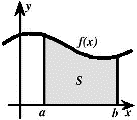## Math in the Middle Institute Partnership## MAT Exam Expository Papers

#### Title

Distance, Rate, Time and Beyond

#### Date of this Version

7-2007

In partial fulfillment of the requirements for the Master of Arts in Teaching with a Specialization in the Teaching of Middle Level Mathematics in the Department of Mathematics. David Fowler, Advisor July 2007

#### Abstract

In middle school mathematics, students learn to use the formula “distance equals rate times time,” usually expressed as d = r × t. Why not consider the formula distance = velocity × time? Does the term velocity mean something different than the term rate? We could also consider the variations of these formulas: distance ÷ time = rate, or distance ÷ rate = time. We can examine the definitions of these words and words which are very similar. After looking at the definitions of these words, maybe we will have a better understanding of how to use the formulas and of the meaning behind them.

COinS